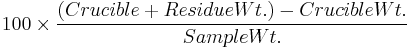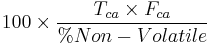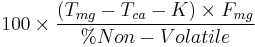106.3.2.27 TM-27, Determination of Calcium Oxide and Magnesium Oxide in Hydrated Lime

This method determines the non-volatile content of hydrated lime and the percentages of calcium oxide and magnesium oxide in that non-volatile.

106.3.2.27.1 Reagents and Apparatus

(a) Platinum Crucible, 20-30 ml volume, with cover

(b) Reagents and Apparatus as described in EPG 106.3.2.26 TM-26, Determination of Calcium Carbonate and Magnesium Carbonate in Lime and Pigment Materials.

106.3.2.27.2 Procedure for Non-Volatile

Weigh, to the nearest 0.1 mg, 1 g of the sample, and transfer to a tarred platinum crucible. Cover the crucible and place in an electric muffle furnace. Bring the furnace to a temperature of 1100-1150°C, and maintain at that temperature for about 15 minutes. Cool the crucible and residue in a dessicator, and weigh to the nearest 0.1 mg.

106.3.2.27.3 Calculations

Calculate the non-volatile as follows:

% Non-Volatile =$100 \times \frac {(Crucible + Residue Wt.)- Crucible Wt.}{Sample Wt.}$

106.3.2.27.4 Procedure for Calcium Oxide and Magnesium Oxide

Determine the calcium and magnesium in the hydrated lime by the procedure described in EPG 106.3.2.26 TM-26, Determination of Calcium Carbonate and Magnesium Carbonate in Lime and Pigment Materials.

106.3.2.27.5 Calculations

Make the following calculations:

% CaO =$100 \times \frac {T_{ca} \times F_{ca}}{% Non-Volatile}$

Report as:

% Calcium Oxide (CaO), based on Non-Volatile
MgO =$100 \times \frac {(T_{mg} - T_{ca} - K) \times F_{mg}}{% Non-Volatile}$

Report as:

% Magnesium Oxide (MgO), based on Non-Volatile